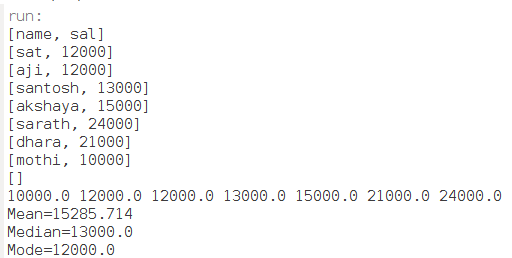• #5, First Floor, 4th Street Dr. Subbarayan Nagar Kodambakkam, Chennai-600 024 Landmark : Samiyar Madam
• pro@slogix.in
• +91- 81240 01111

#### Description

To calculate the measure of dispersion for a sample data set in java.

###### Process
Mean:

It is calculated by taking the sum of the values and dividing with the number of values in a data series.

Median:

The middle most occurrence value in a data series is called the median.

Mode:

The mode is the value that has highest number of occurrences in a set of data.

###### Sample Code

package Stat;

import java.io.File;
import java.io.FileNotFoundException;
import java.io.IOException;
import java.io.PrintWriter;
import java.util.ArrayList;
import java.util.Collections;
import java.util.Scanner;

public class mean {
public static void main(String[] args) throws FileNotFoundException, IOException {

PrintWriter out = new PrintWriter(new File(“file2.csv”));
File file = new File(“file2.csv”);

int skipIndex = 1;
String line1;
while ((line1 = in.readLine()) != null) {
String[] parts = line1.split(” “);
StringBuilder outLine = new StringBuilder();
for (int i = 0; i < parts.length; i++) {
if (i + 1 != skipIndex) {
outLine.append(” ” + parts[i]);
}
}

out.println(outLine.toString().trim());

}

in.close();
out.close();

String line;

Scanner sc = null;

try {
sc = new Scanner(file);
sc.next();
} catch (FileNotFoundException e) {
e.printStackTrace();
return;
}

ArrayList list = new ArrayList();

while (sc.hasNextFloat())

int size = list.size();

if (size == 0) {
System.out.println(“Empty list”);
return;
}

Collections.sort(list);

for (int i = 0; i < size; i++) {
System.out.print(list.get(i) + ” “);
}

System.out.println();

// mean value
float sum = 0;
for (float x : list) {
sum += x;
}

float mean = sum / size; // mean as integer

//median
float median;

if (size % 2 == 0) {
float x1 = list.get(size / 2 – 1);
float x2 = list.get(size / 2);
median = (x1 + x2) / 2;
} else {
median = list.get(size / 2);
}

//mode
Float mode = null;
int counter = 1;
for (int i = 0; i < size; i++) { int freq = Collections.frequency(list, list.get(i)); if (freq > counter) {
mode = list.get(i);
counter = freq;
}
}
System.out.println(“Mean=” + mean);

System.out.println(“Median=” + median);
if (mode == null) {
System.out.println(“No mode found”);
} else {
System.out.println(“Mode=” + mode);
}
}
}

###### Screenshots